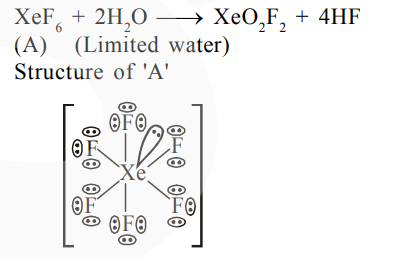# A xenon compound 'A' upon partial hydrolysis`
Question:

A xenon compound 'A' upon partial hydrolysis gives $\mathrm{XeO}_{2} \mathrm{~F}_{2}$. The number of lone pair of electrons present in compound $A$ is_________ (Round off to the Nearest integer)

Solution:Total l.p. on $(\mathrm{A})=19$